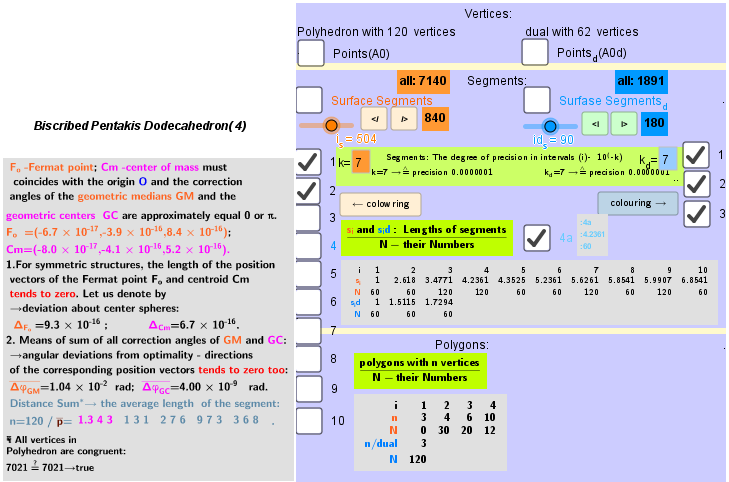# Images . Truncated icosidodecahedron (V=120) from Biscribed Pentakis Dodecahedron for the case of trisection of its 4th-order segments

Generating Elements of mesh modeling the surfaces of polyhedron, its dual image and the coloring of their edges and faces can be found in the applet﻿.The elements of the Biscribed Pentakis Dodecahedron(4) -Truncated icosidodecahedron. It bounded by: Vertices: V =120. Faces: F =62. 30{4}+20{6}+12{10} Edges: E =180. 60+60+60 - The order of the number of edges in this polyhedron are according to their length.
Truncated icosidodecahedron: https://en.wikipedia.org/wiki/Truncated_icosidodecahedron
 Type Archimedean solid Uniform polyhedron Elements F = 62, E = 180, V = 120 (χ = 2) Faces by sides 30{4}+20{6}+12{10}
The elements of the dual to the Biscribed Pentakis Dodecahedron(4) (Truncated icosidodecahedron) -Disdyakis triacontahedron Vertices: V = 62. Faces: F =120. 120{3} Edges: E =180. 60+60+60- the order of the number of edges in this polyhedron are according to their length.
 Disdyakis triacontahedron :
 Type Catalan Conway notation mD or dbD Coxeter diagramFace polygonscalene triangle Faces 120 Edges 180
https://en.wikipedia.org/wiki/Disdyakis_triacontahedron